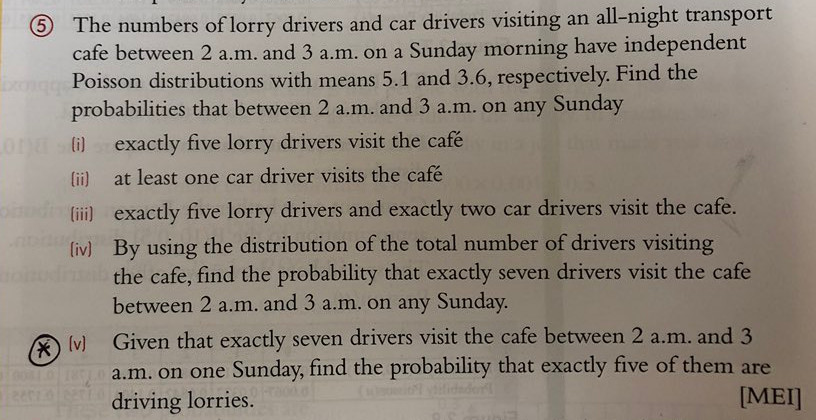# Julian's musings

## A curious Poisson distribution question

Yvonne Scott posted the following question:Stuart Price noted that the answer to the last part can be obtained as the answer to part (iii) divided by the answer to part (iv), by the definition of conditional probability.

But if we think about what’s going on a little further, we will be able to understand the structure of this problem more and see further connections.

The first thing to do to make our life a little simpler is to replace the specific numbers 5.1 and 3.6 with variables, so that the algebraic structure becomes clearer. So let’s call the means of the two independent Poisson distributions $\lambda$ and $\mu$. We will stick with the 5 and 7 for the time being, and generalise those later.

Therefore our problem says that the number of lorry drivers is distributed as $\mathrm{Po}(\lambda)$, the number of car drivers is distributed as $\mathrm{Po}(\mu)$ and the total number of drivers is distributed as $\mathrm{Po}(\lambda+\mu)$. The relevant probabilities are then as follows:

\begin{align*} \mathrm{P}(\text{5 lorry drivers}) &= \dfrac{e^{-\lambda}\lambda^5}{5!}\\ \mathrm{P}(\text{2 car drivers}) &= \dfrac{e^{-\mu}\mu^2}{2!}\\ \mathrm{P}(\text{7 drivers}) &= \dfrac{e^{-(\lambda+\mu)}(\lambda+\mu)^7}{7!}\\ \mathrm{P}(\text{5 lorry drivers} \mid \text{7 drivers}) &= \dfrac{\mathrm{P}(\text{5 lorry drivers and 2 car drivers})} {\mathrm{P}(\text{7 drivers})}\\ &= \dfrac{\frac{e^{-\lambda}\lambda^5}{5!}\times \frac{e^{-\mu}\mu^2}{2!}} {\frac{e^{-(\lambda+\mu)}(\lambda+\mu)^7}{7!}}\\ &= \dfrac{\lambda^5\mu^2\times 7!}{(\lambda+\mu)^7\times 5!2!}\\ &= \dbinom{7}{5}\left(\dfrac{\lambda}{\lambda+\mu}\right)^5 \left(\dfrac{\mu}{\lambda+\mu}\right)^2 \end{align*}

But this is just a binomial probability! It is the probability of 5 successes from 7, where the probability of success is $\frac{\lambda}{\lambda+\mu}$, which equals the mean number of lorry drivers divided by the mean number of drivers. It is clear that we could replace 5 and 7 by any numbers $r$ and $n$ in the above calculation, to deduce that given that there are $n$ drivers in total, the probability that $r$ of them are lorry drivers is

$\dbinom{n}{r}\left(\dfrac{\lambda}{\lambda+\mu}\right)^r \left(\dfrac{\mu}{\lambda+\mu}\right)^{n-r}.$

If we had assumed that the probability that a visiting driver picked at random is a lorry driver is $\frac{\lambda}{\lambda+\mu}$, then we would have got the same answer without having to calculate any Poisson probabilities at all.

This seems like a reasonable suggestion, but how can we justify it?

One technical way is to say that the binomial probabilities we have found above prove that this is the case. But this gives little insight into the reason for it.

A better way is to simply observe that the ratio of the rate of lorry drivers arriving to the rate of car drivers arriving is $\lambda:\mu$, so the probability that a particular driver is a lorry driver is indeed $\frac{\lambda}{\lambda+\mu}$. This might feel a little problematic, though, as it seems to ignore the probabilistic aspects involved.

A more careful way of doing this is to think about the behaviour and meaning of Poisson distibutions. The means $\lambda$ and $\mu$ are for the unit time period of 1 hour. If we had a time period of $t$ hours, with the same uniform random driver arrivals over the whole period, then the mean number of lorry and car drivers would be $t\lambda$ and $t\mu$ respectively, with the distribution of the number of drivers still being Poisson. A standard thing to do at this point is to take $t$ to be very small. In this case, the probability of there being more than one driver arriving in the period is negligible, so the probabilities become:

\begin{align*} \mathrm{P}(\text{lorry driver arrives}) &= t\lambda\\ \mathrm{P}(\text{car driver arrives}) &= t\mu\\ \mathrm{P}(\text{no driver arrives}) &= 1-t\lambda-t\mu \end{align*}

Two ways of deriving these probabilities are: (a) calculate the Poisson probabilities, expanding $e^{-t\lambda}$ and ignoring all terms involving $t^2$; (b) assume that the number of lorry drivers arriving is zero or one, then calculate what the probability of one lorry driver arriving would have to be so that the expected number of lorry drivers is $t\lambda$. Note that we also ignore the negligible probability that both a lorry driver and car driver arrive.

Therefore, in this very short time period of $t$ hours, we have

$\mathrm{P}(\text{lorry driver arrives}\mid \text{any one driver arrives}) = \frac{t\lambda}{t\lambda+t\mu} = \frac{\lambda}{\lambda+\mu}.$

This means that whenever a driver arrives, the probability that this driver is a lorry driver is indeed $\frac{\lambda}{\lambda+\mu}$, exactly as we wanted.

Incidentally, the small-time-slice thinking also shows why the Poisson distribution is a good approximation to the binomial distribution: imagine we are dealing with a time interval and our Poisson distribution has mean $\lambda$. Divide the whole time interval into $N$ equal slices, and assume that no slices can have more than one event. Then each slice has a probability of $\lambda/N$ of having an event, and the number of events is distributed as $\mathrm{B}(N,\lambda/N)$. The larger $N$ is, the better the assumption that no slice can have more than one event becomes, and so the more closely $\mathrm{B}(N,\lambda/N)$ approximates $\mathrm{Po}(\lambda)$.

This also reminds me of a lovely and surprising probability question on this topic that I saw on an undergraduate problem set (question 6(ii) on Examples sheet 2 here):

The number of misprints on a page has a Poisson distribution with parameter $\lambda$, and the numbers on different pages are independent. A proofreader studies a single page looking for misprints. She catches each misprint (independently of others) with probability 1/2. Let $X$ be the number of misprints she catches. Find $\mathrm{P}(X=k)$. Given that she has found $X=10$ misprints, what is the distribution of $Y$, the number of misprints she has not caught? How useful is $X$ in predicting $Y$?